# Degrees Fahrenheit

$C=\frac{5}{9}\left(F\text{−}32\right)$

The equation above shows how temperature F, measured in degrees Fahrenheit, relates to a temperature C, measured in degrees Celsius. Based on the equation, which of the following must be true?

I. A temperature increase of 1 degree Fahrenheit is equivalent to a temperature increase of 5/9 degree Celsius.
II. A temperature increase of 1 degree Celsius is equivalent to a temperature increase of 1.8 degrees Fahrenheit.
III. A temperature increase of 5/9 degree Fahrenheit is equivalent to a temperature increase of 1 degree Celsius.

A) I only
B) II only
C) III only
D) I and II only

Result

x = (Correct answer is: D)### Step-by-step explanation:

$x=D$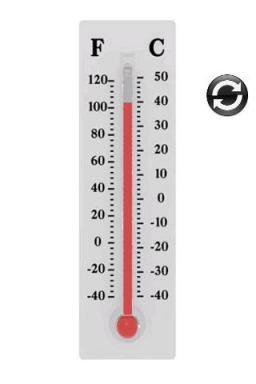Did you find an error or inaccuracy? Feel free to write us. Thank you!Tips to related online calculators
Need help to calculate sum, simplify or multiply fractions? Try our fraction calculator.

## Related math problems and questions:

• Mixing Celsius and FahrenheitAdd up three temperatures: 5°F +6°F +0°C
• Temperature calculus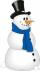Calculate the percentage of temperature increase if changed from 18°C to 33°C?
• Change in temperatureStarting temperature is 21°C, the highest temperature is 32°C. What is the change in temperature?
• Freezer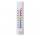The temperature is -8 degrees Celsius in the freezer. The outside temperature is 2 degrees Celsius. One minute took to change the temperature by one degree Celsius if we open the door. How many minutes the temperature in the freezer will rise?
• Heat transferWe placed a lead object weighing 0.4 kg and 250°C in 0.4 L water. What was the initial temperature of water t2 if the object's temperature and the water after reaching equilibrium was 35°C? We assume that the heat exchange occurred only between the lead o
• Fractions and mixed numerals(a) Convert the following mixed numbers to improper fractions. i. 3 5/8 ii. 7 7/6 (b) Convert the following improper fraction to mixed number. i. 13/4 ii. 78/5 (c) Simplify these fractions to their lowest terms. i. 36/42 ii. 27/45 2. evaluate following ex
• The temperature 13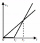The temperature in Toronto at noon during a winter day measured 4°C. The temperature started dropping 2° every hour. Which inequality can be used to find the number of hours, x, after which the temperature will measure below -3°C?
• Temperature difference 2The temperature in London on new year’s day is -2 degree Celsius. The temperature in Moscow on the same day is -14 degree Celsius, what is the temperature difference between the two cities?
• Thermometer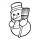The thermometer showed -3°C in the morning. Then the temperature was increased by 1°C again increased by 1°C and then decreased by 1°C and then decreased by 4°C. Which terminal temperature thermometer shows?
• Daily temperature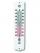The average of daily temperature measurements in one week every day at the same hour was -2.8 °C. We measured all temperatures on different days are different. The highest daily maximum temperature was 2.4 °C, the lowest -6 °C. Determine the options that
• The poolThe pool has a volume of 40 m3, and the water temperature is 20 °C. How much water at 100 °C should we pour into the pool to increase the water temperature by 5 °C?
• Bath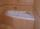In the bath is 30 liters of hot water. Then added 36 liters of cold water at temperature of 19 °C decreased temperature of water to 41 °C. What was the initial temperature of the hot water?
• Electrics - conductor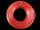The wire is 106 meters long at 0 °C and at every temperature increase of 1 °C the length increases by 0.15 mm per 1 m length of wire. Determine a function which represents the overall length of the wire as a function of temperature. What is the length of
• Latent heat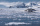How much heat is needed to take from 100 g of water at 20°C to cool to ice at -18°C? Mass heat capacity c (ice) = 21kJ/kg / °C; c (water) = 4.19 KJ/kg / °C and the mass group heat of solidification of water is l = 334kJ/kg
• Thermometer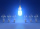Immerse a thermometer with a heat capacity of 2.0 J. K-1 in water weighing 67.0 grams. Before immersion in water, the thermometer showed a temperature of 17.8 degrees Celsius. After reaching equilibrium, the temperature is 32.4 degrees. What was the water
• Average temperatureThe average temperature from Monday to Sunday was 40.5°F and the average temperature from Monday to Saturday was 42.8°F. What was the temperature on Sunday?
• The bathDad poured 50 liters of water at 60°C into the tub. How much cold water must be added at 10°C to obtain a bath that has a temperature of 40°C?